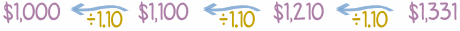# Investment/Loan Graph

Try different values, periods and interest rate to see how your loan or investment will perform.
Explanations Below!

(The old flash version is available here.)

## Explanations

At 10% Interest money grows by 10% every year (as explained in Compound Interest):Which is easier to calculate this way (same result):## Present Value (PV)

To see what money in the future is worth now, go backwards (dividing by 1.10 for 10% Interest):### Example: Sam promises you \$500 next year, what is the Present Value?

To take a future payment backwards one year divide by 1.10

So \$500 next year is \$500 ÷ 1.10 = \$454.55 now (to nearest cent).

The Present Value is \$454.55

See a detailed explanation at Present Value.

## Net Present Value (NPV)

The Net Present Value is the total of all Present Values, including subtracting money that went out (the money you invested or spent):

• Subtract the Present Values you pay

### Example: You invest \$500 now, and get back \$570 next year (interest Rate is 10%)

Money Out: \$500 now

You invested \$500 now, so PV = -\$500.00

Money In: \$570 next year

PV = \$570 / (1+0.10)1 = \$570 / 1.10 = \$518.18 (to nearest cent)

And the Net Amount is:

Net Present Value = \$518.18 - \$500.00 = \$18.18

So, at 10% interest, that investment is worth \$18.18

A Net Present Value (NPV) that is positive is good (and negative is bad).

Read more at Net Present Value